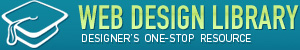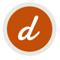SEARCH
Tutorials HTML and CSS Tutorials Creating A Web Page Calculator

# Creating A Web Page Calculator

Provided by developerdrive.com

HTML5 includes a host of new input elements, such as the output tag. Using the output tag in conjunction with the "oninput" event listener, you can create simple or complex Web page calculators, giving the user instant results. The output tag allows you to build forms using semantic markup, since the element is specifically designed for displaying calculation results.

In this tutorial we will create a simple Web page calculator to demonstrate using the output element. Many of the new input elements are not well supported, but the output element is supported in all current major browsers except Internet Explorer. We will also be using the "oninput" event attribute, which is supported in all recent versions of the main browsers.

## Create an HTML5 Page

Use the following outline to create your HTML5 page:

```<!DOCTYPE html>
<html>
<style type="text/css">

</style>
<script type="text/javascript">

</script>
<body>

</body>
</html>```

We have sections for styling and scripting in the head, and our form elements will sit within the body.

## Create a Form

```<form id="calc" oninput="updateOutput()">
<!--input and output elements-->
</form>```

Our opening form tag has an ID for identification in JavaScript and an "oninput" event listener attribute. The browser will call the specified function when the user alters the form input values.

```<input name="x" type="number" value="0" />

<input name="y" type="number" value="0" />```

Leave a space between these as we are going to add a further element next. Notice that the two input elements have number types and names for identification in the script.

Let's allow users to choose which operator they wish to use in their calculation. Add a select element between the two number input elements:

```<select name="op" onchange="updateOutput()">
<option value="0">+</option>
<option value="1">-</option>
<option value="2">x</option>
<option value="3"> </option>
</select>```

You can use the HTML entity references "&times;" and "&divide;" for your multiplication and division signs.

The select element offers addition, subtraction, multiplication and division. To make sure the output element is updated when the user chooses an option, as well as when they alter the number input values, we add the "onchange" event listener attribute, calling the same function we call from the form "oninput" attribute. We are using incrementing integers as the values for the select options, so that we can tailor what happens each time the calculation is performed, making sure the output reflects the operator chosen.

Add an equals sign in a dedicated element so that we can style it effectively, after the second number input:

`<div class="equals"> = </div>`

Finally, let's add our output element, after the equals sign element and still inside the form:

`<output name="z" for="x y">0</output>`

Notice that the element has a name for identification in the script and a default value to display. The "for" attribute indicates which input elements the output relates to, using their name attributes.

## Perform the Calculation

Now we can implement the JavaScript function for calculating results. In the script section in your page head, add the following function outline:

```function updateOutput() {
//calculate

}```

Inside the function, first retrieve a reference to the form element:

```	//get form
var form = document.getElementById("calc");```

```	//get output
var out = form.elements["z"];```

Retrieve the current values in each input element as numbers:

```	//get two numbers
var num1 = parseInt(form.elements["x"].value);
var num2 = parseInt(form.elements["y"].value);```

Note: the "valueAsNumber" property can be used instead of parsing input as integers as we have done here, but the technique doesn't yet enjoy full browser support.

Let's now find out which operator the user has selected:

```	//get operator
var operator = parseInt(form.elements["op"].value);```

Remember that we simply used integer values for the select options, so we can use a switch statement to tailor the output:

```	//set output depending on operator
switch(operator)
{
case 0:
out.value = num1+num2;
break;
//subtract
case 1: out.value = num1-num2;
break;
//multiply
case 2: out.value = num1*num2;
break;
//divide
case 3: out.value = (num1/num2).toFixed(1);//only one digit after decimal place
break;
default:
break;
}```

The calculation is tailored to the chosen operator. Notice also that with division we are opting to output a particular precision, so that only one digit will be displayed following the decimal point.

## Style the Calculator

This is of course optional, but let's style the calculator - here is how it looks in Firefox:In certain browsers the number inputs will appear with arrows so that the user can increment and decrement the values without having to manually type them. This is the calculator in Chrome:And it's different again in Opera:The elements appear pretty differently across browsers, which is worth bearing in mind when working on your page designs. In your head style section, add the following declarations which you can naturally alter:

```/*number inputs*/
input[type="number"] {
width:50px; height:30px;
text-align:center;
margin:3px;
float:left;}

/*select and equals elements*/
select, .equals {
margin:3px;
float:left;}

/*output element*/
output {
display:block;
border:1px solid #333333;
min-width:25px; height:25px;
text-align:center;
background:#000000;
color:#ffffff;
float:left; }```

That's our basic calculator complete, so open your page in a supporting browser to test it.

## Conclusion

Like many HTML5 developments, the output element does not introduce particularly new functionality to your pages, but alters the ways in which you can achieve certain results. By choosing a semantic element, your pages will have a level of meaning built into their structures.